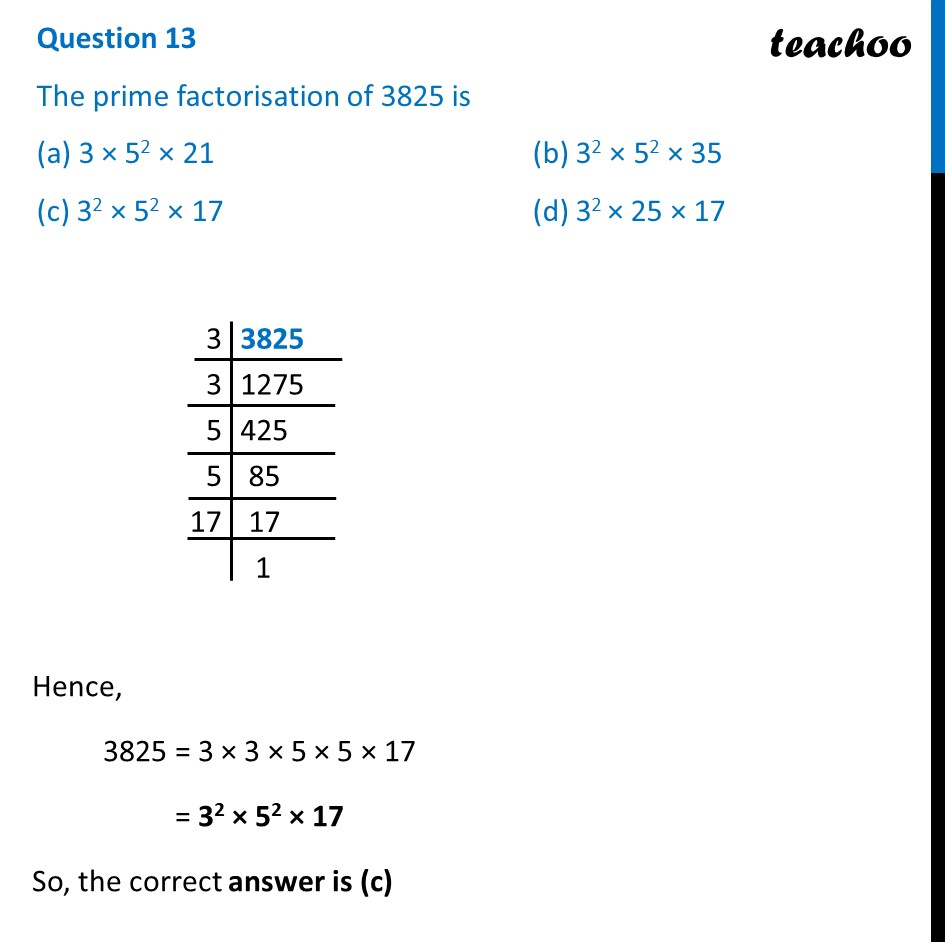## (c) 3 2 × 5 2 × 17   (d) 3 2 × 25 × 17

This question is inspired from Ex 1.2, 1 (iii) - Chapter 1 Class 10 - Real Numbers1. Class 10
2. Solutions of Sample Papers for Class 10 Boards
3. CBSE Class 10 Sample Paper for 2022 Boards - Maths Basic [MCQ]

Transcript

Question 13 The prime factorisation of 3825 is (a) 3 × 52 × 21 (b) 32 × 52 × 35 (c) 32 × 52 × 17 (d) 32 × 25 × 17 Hence, 3825 = 3 × 3 × 5 × 5 × 17 = 32 × 52 × 17 So, the correct answer is (c)